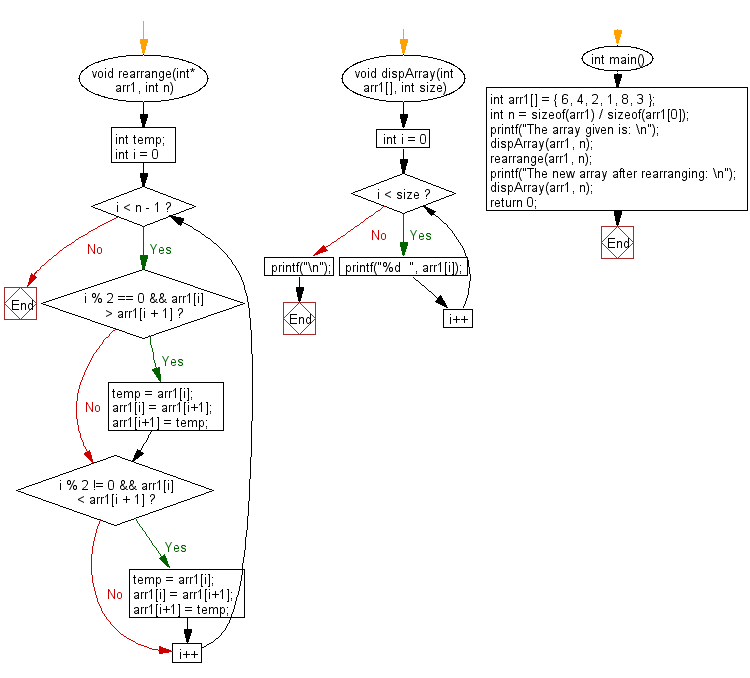﻿ C exercises: Rearrange an array such that even index elements are smaller and odd index elements are greater than their next - w3resource# C Exercises: Rearrange an array such that even index elements are smaller and odd index elements are greater than their next

## C Array: Exercise-104 with Solution

Write a program in C to rearrange an array such that even index elements are smaller and odd index elements are greater than their next.

Sample Solution:

C Code:

``````#include<stdio.h>
void rearrange(int* arr1, int n)
{
int temp;
for (int i = 0; i < n - 1; i++)
{
if (i % 2 == 0 && arr1[i] > arr1[i + 1])
{
temp = arr1[i];
arr1[i] = arr1[i+1];
arr1[i+1] = temp;
}
if (i % 2 != 0 && arr1[i] < arr1[i + 1])
{
temp = arr1[i];
arr1[i] = arr1[i+1];
arr1[i+1] = temp;
}
}
}

void dispArray(int arr1[], int size)
{
for (int i = 0; i < size; i++)
printf("%d  ", arr1[i]);
printf("\n");
}

int main()
{
int arr1[] = { 6, 4, 2, 1, 8, 3 };
int n = sizeof(arr1) / sizeof(arr1);
printf("The array given is: \n");
dispArray(arr1, n);
rearrange(arr1, n);
printf("The new array after rearranging: \n");
dispArray(arr1, n);
return 0;
}
```
```

Sample Output:

```The array given is:
6  4  2  1  8  3
The new array after rearranging:
4  6  1  8  2  3
```

Pictorial Presentation:Flowchart:C Programming Code Editor:

Improve this sample solution and post your code through Disqus.

﻿